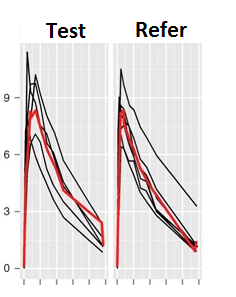## R script published pubmed site not work [🇷 for BE/BA]

Dear Friends,

I would like to understand why the code bellow is not working. This code I was take out on the page: https://www.ncbi.nlm.nih.gov/pmc/articles/PMC3817376/. Would it be possible for any BEBAC member to adapt it to work? I need of the a graphics tool (for example: ggplot2) that to make graphics dinamic way as we can make at Phoenix WinNonlin.

(ggplot2) library(nlme) head(Theoph) ggplot(data=Theoph, aes(x=Time, y=conc, group=Subject)) + geom_line() + labs(x=“Time (hr)”, y=“Concentration (mg/L)”) p <- ggplot(data=Theoph, aes(x=Time, y=conc, group=Subject)) + geom_line() + labs(x=“Time (hr)”, y=“Concentration (mg/L)”) + stat_summary(fun.y=median, geom=“line”,aes(x=ntpd, y=conc, group=1), color=“red”, size=1) print(p) # “p” is a ggplot object # create a flag for body weight Theoph$WT <- ifelse(Theoph$Wt<70, “WT < 70kg”, “WT >= 70kg”) p + facet_grid(.~WT)""t> 

In true, I need of a dinamic code (for example: ggplot2) for to plot a graphic of Individual plasma concentration profiles sorted by treatment and period with whih the mean. Example:Ing. Helmut Schütz